# Trigonometry Lesson Plan Of Mathematics For B.Ed/DELED And School Teachers

0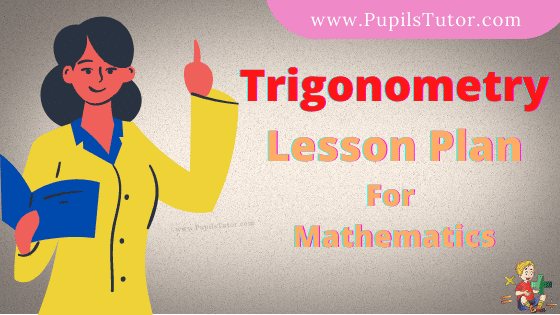Hello Friends, Welcome To Our Website. How Are You? Hope You Are Doing Well,

If You Are Searching For Trigonometry Lesson Plan Then You Have Come To The Right Place. Here We Have Shared The Lesson Plan On Trigonometry For B.Ed, D.El.Ed, And School Teachers.

This Mega And Real School Teaching Math Lesson Plan On Trigonometry Is Specially Made For The B.Ed 1st And 2nd Year Students But All The Trainee Teacher And School Teachers Of All Classes Can Prepare Their Final Teaching Plan Very Easily With The Help Of This Sample Trigonometry Lesson Plan For Class 9 To 12.

Brief Overview Of The Lesson Plan

 Class 10th, 11th Topic Trigonometry Subject Mathematics Lesson Plan Type Real School Teaching And Practice Skill School Teaching

The Topics And Points That Are Covered In This Mathematic Lesson Plan On Trigonometry Are : Trigonometry, Trigonometric Functions, Sine, Cosine, Cosecant, Secant, Tangent, Cotangent, Trigonometric Ratio, Relationship Between Trigonometric Ratio, Identities In Trigonometry, Trigonometric Ratio Of Complementary Angles…...

## Trigonometry Lesson Plan –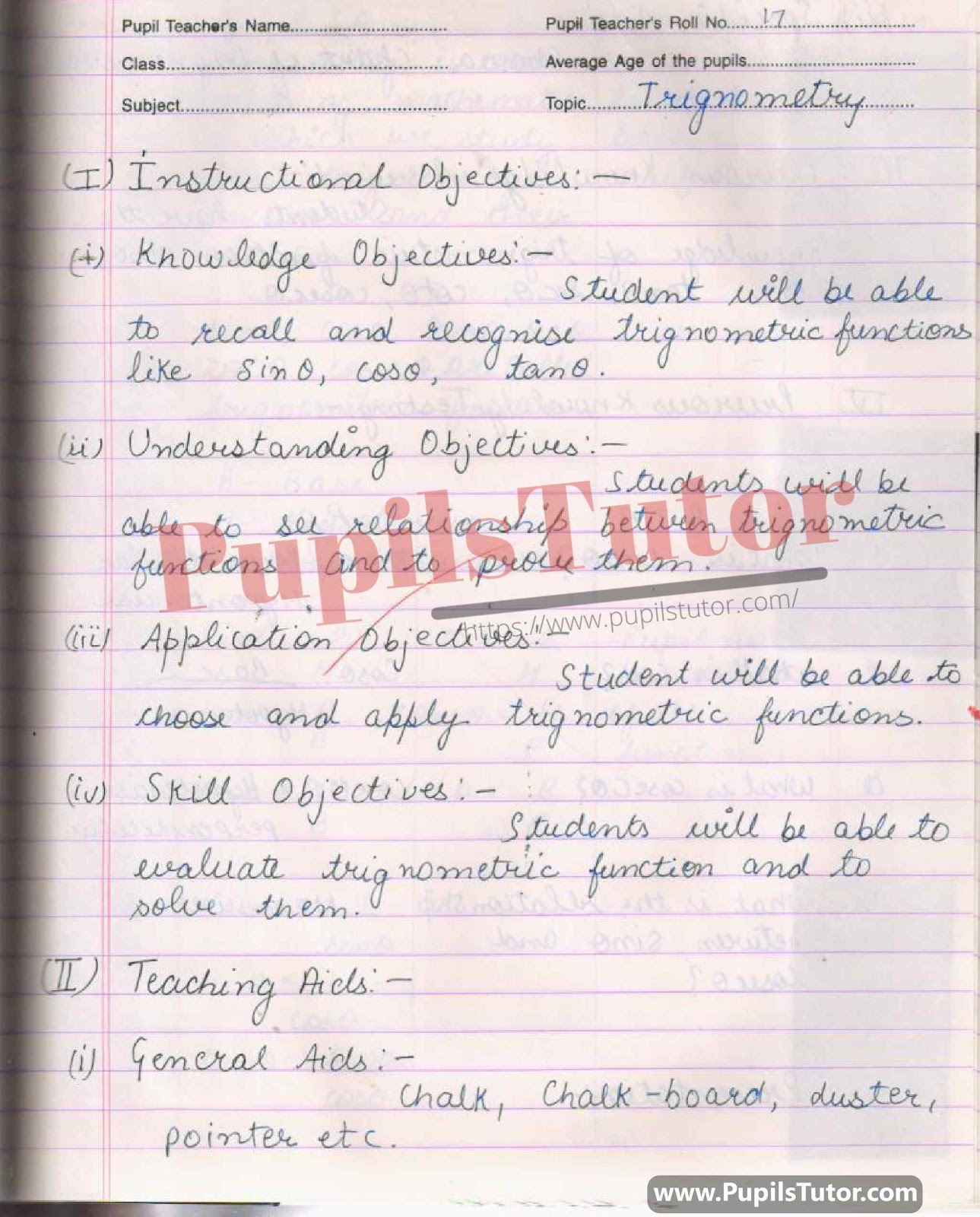## Real School Teaching Trigonometry, Trigonometry identity, Trigonometry Ratios, Angle, Complementary Angles, Trigonometry Function Lesson Plan For B.Ed And Deled In English Free Download PDF And PPT (PowerPoint Presentation And Slides) –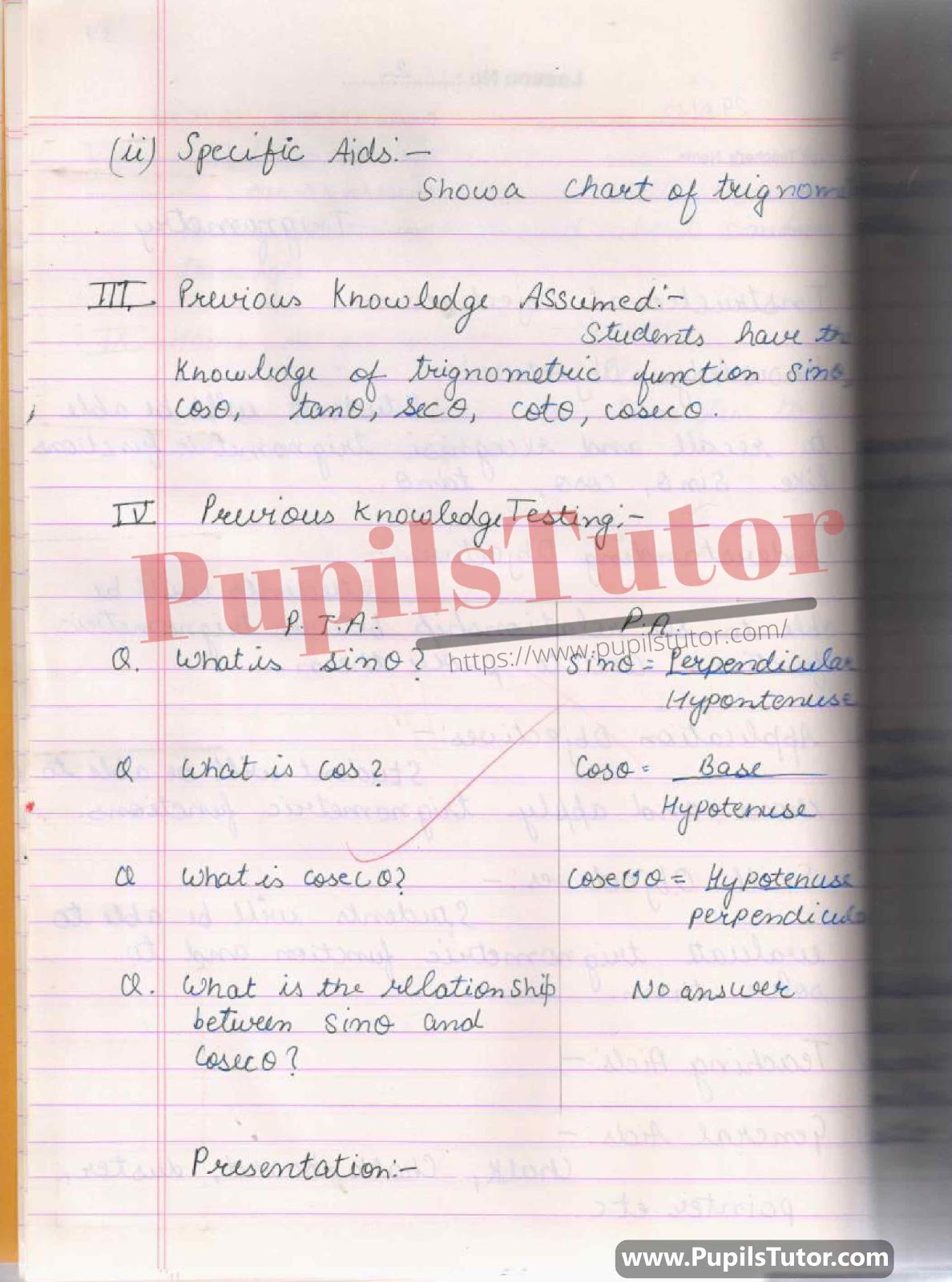## Mathematics Lesson Plan On Sin, Cos, Cot, Tan, Sec, Cot, Trigonometry Formulas, Trigonometry Angles, Trigonometry Table, Sin Cos Identity, Tan Sec Identity, Cosec Sec Identity, Tigonmetry Reverse Formulas, Trigonometry For Class/Grade 9th, 10th, 11th, 12th For CBSE NCERT School And College Teachers –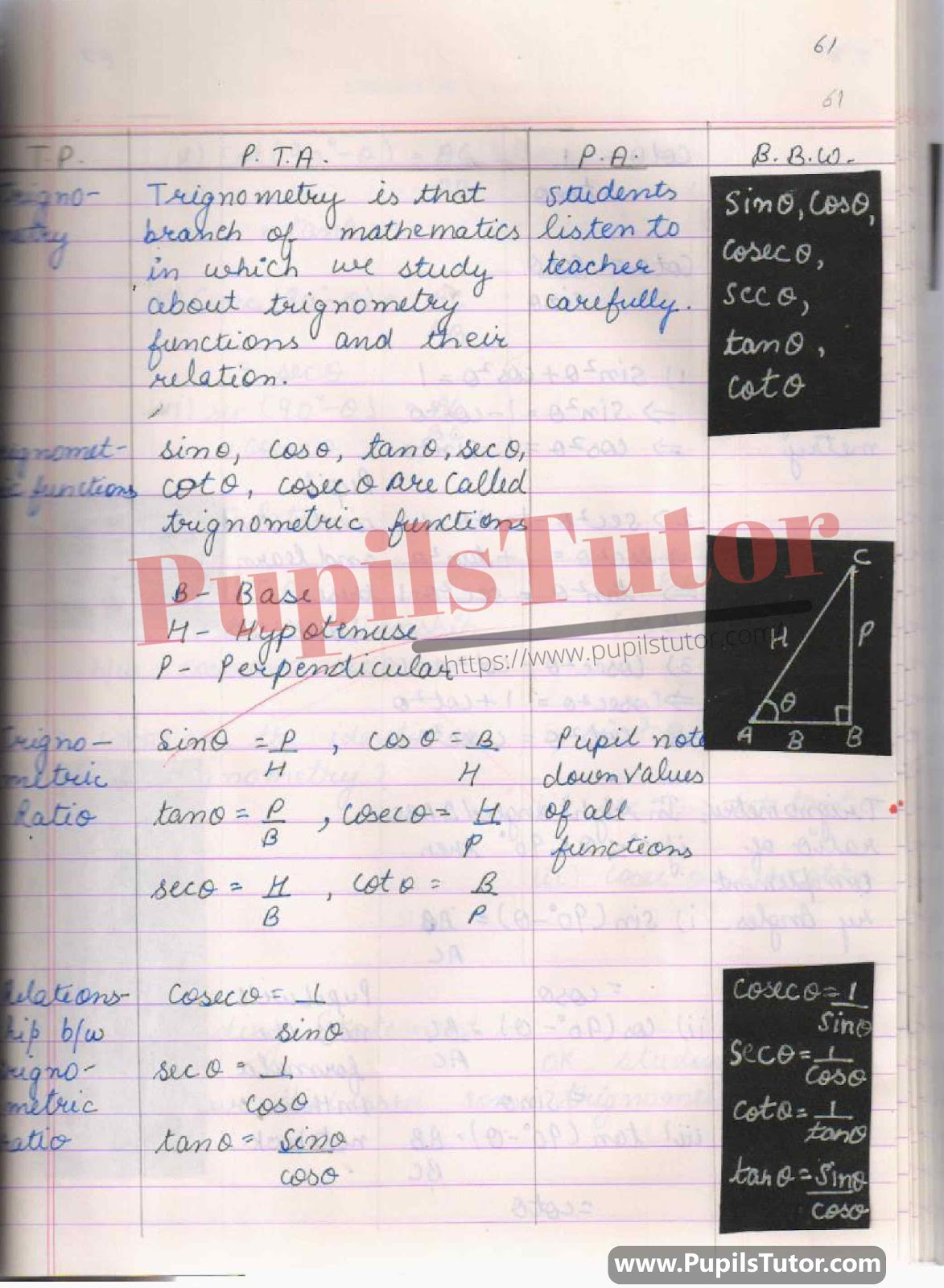## BED, DELED, BTC, BSTC, M.ED, DED And NIOS Teaching Of Math Innovative Digital Lesson Plan Format On Trigno, Perpendicular(P), Base(B), Hypotenuse(H), Right Angle Triangle, Complimentary Trigno Formulas, Trigno Identity, Relationship between Sin And Cosec, Relationship Between Tan And Cot, Relationship Between Cos And Sec Topic For Class 9, 10, 11, 12 –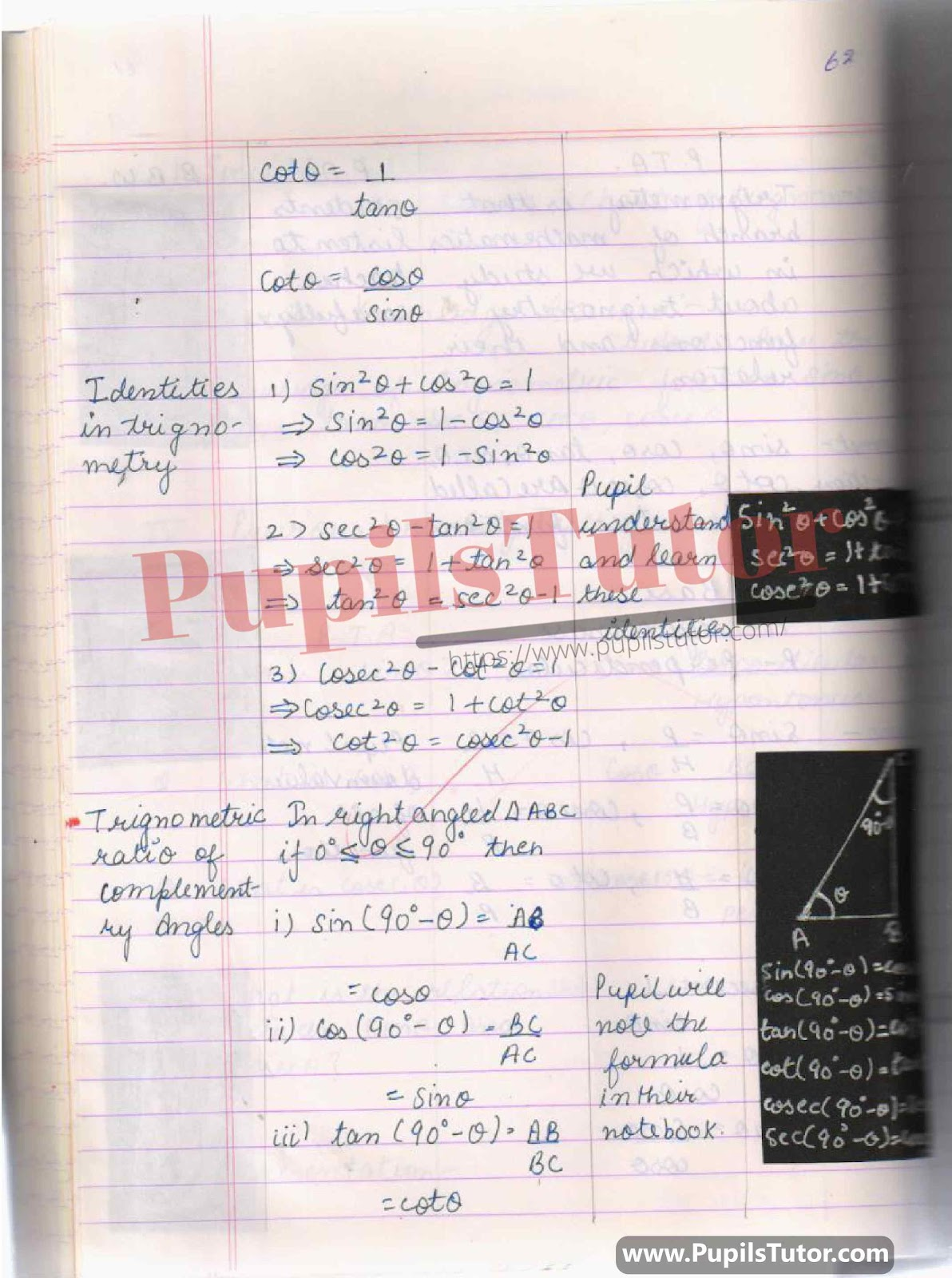## Latest Lesson Plan On Trigonometry, Trigno, Trigno Angle, Trignometry 3 Identities, Trigonometry Relationship, Trigonometry Functions, Relationship Between Cos And Sin, Relationship Between Tan And Sec, Relationship Between Cot And Cosec For Class 10th, 11th. –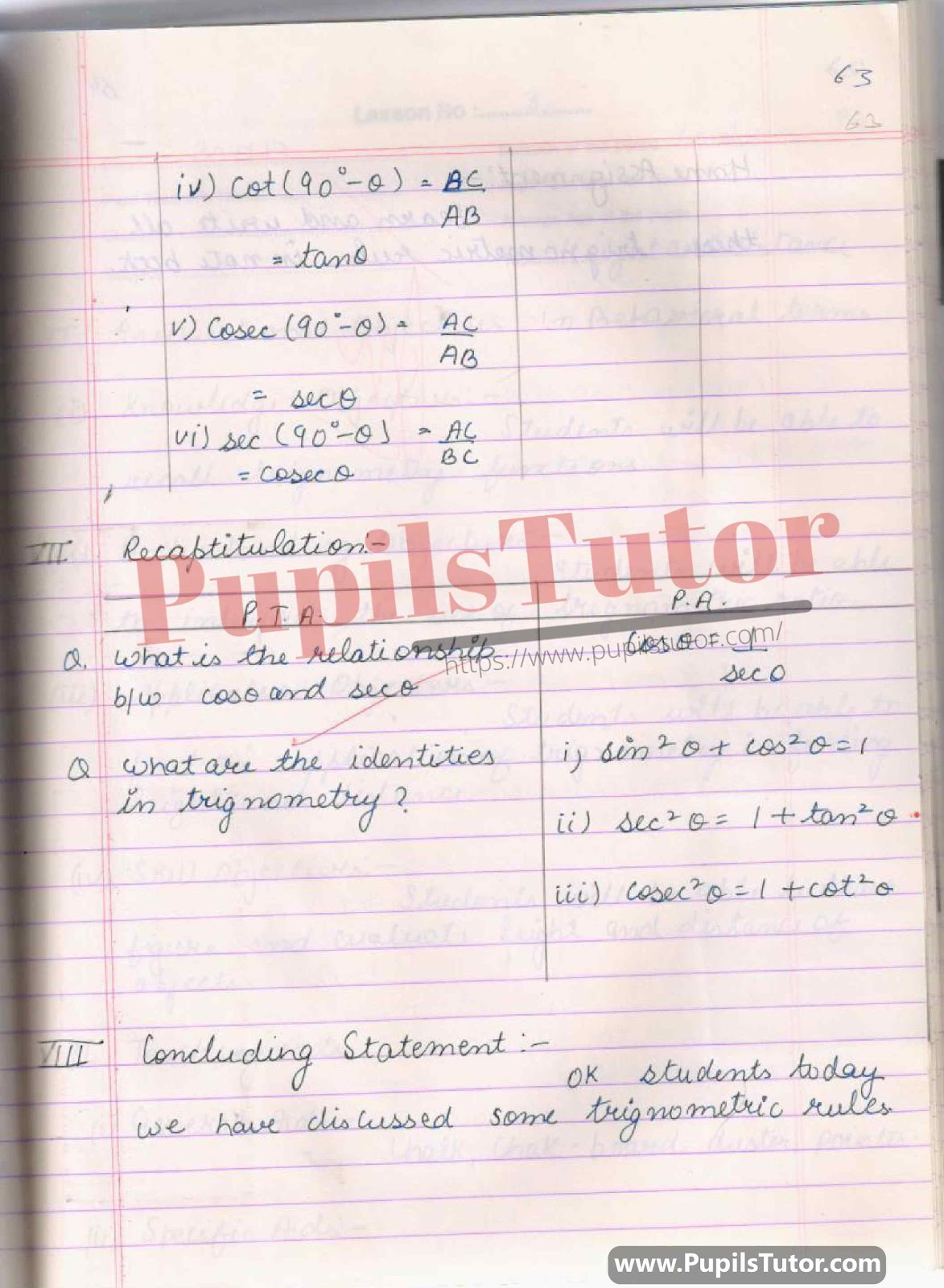## What Is Trigonometry, Trigonometries Formulas, Trigonometry Ratio, Trigonometry Complementary Angle, Relationships Between Trignometry Function And Ratio, Examples, Home Assignment Lesson Plan For B.Ed 1st Year, 2nd Year And All Semesters Students –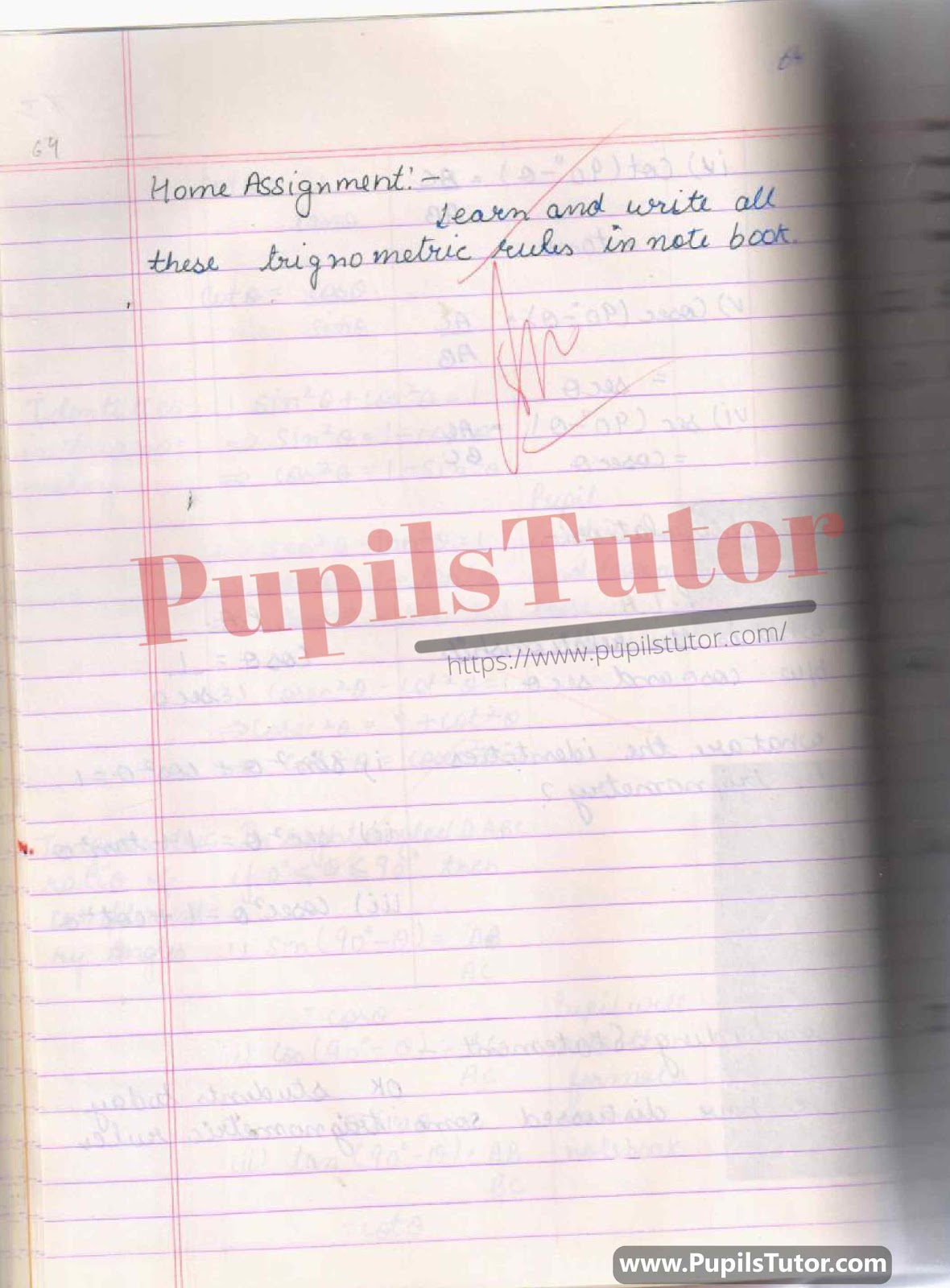You Might Also Like:

Further Reference

 B.Ed. Question Bank And Exam Notes A Complete Guide For B.ED. Entrance Examination CTET & TETs Preparation Material Teaching Aptitude and Attitude Test Book UP B.Ed Guidebook & Latest Practice Sets Book For Entrance Exam B.Ed CET Delhi University B.Ed Entrance Test Latest Practice Sets IGNOU B.Ed. Entrance Test: Previous Years Papers (Solved) Assignments And Files Lesson Planning Files And Guide

On Our Website www.PupilsTutor.Com, We Have Also Shared Lots Of Other Lesson Plans Of All Subjects On Various Teaching Skills Like Micro-Teaching, Mega Teaching, Discussion Skill, Real School Teaching And Practice Skill, Simulated And Observation Skills. You Can Also Check Them.

If You Liked This Trigonometry Lesson Plan For Class 10th, 11 Then Please Share Our Efforts With Your Friends Also.

You Can Also Share Your Lesson Plans, Assignments, Files, Papers And Study Notes With Us To Help Other Students .

#### List Of Some More Mathematics Lesson Plans

 Profit And Loss B.ed Maths Lesson Plan Sum Of The Angles Of A Triangle B.ed Maths Lesson Plan Trigonometric Ratio B.ed Maths Lesson Plan Circle Bed Maths Lesson Plan Properties Of Angle In Circle B.ed Maths Lesson Plan Pie Chart B.ed Maths Lesson Plan Three Dimensional Shape B.ed Maths Lesson Plan Trigonometry B.ed Maths Lesson Plan Parts Of The Circle B.ed Maths Lesson Plan Compound Interest B.ed Maths Lesson Plan Volume Of A Cone And Cylinder B.ed Maths Lesson Plan Areas Related To Circle B.ed Maths Lesson Plan Integer B.ed Maths Lesson Plan Surface Area Of Cone And Cylinder B.ed Maths Lesson Plan Statistics And Arithmetic Mean B.ed Maths Lesson Plan Volume Of Right Circular Cylinder B.ed Maths Lesson Plan Circular Trigonometry Function B.ed Maths Lesson Plan 3D Shapes B.ed Maths Lesson Plan Coordinate Geometry Section Formula B.ed Maths Lesson Plan Surface Area And Volume Of Sphere B.ed Maths Lesson Plan Theorem B ed Maths Lesson Plan Curved And Total Surface Area Of Cylinder Bed Maths Lesson Plan Quadrilateral B.ed Maths Lesson Plan Height And Distance B.ed Maths Lesson Plan Curved And Total Surface Area Of Cone B.ed Maths Lesson Plan Coordinate Geometry B.ed Maths Lesson Plan Similar Triangle B.ed Maths Lesson Plan Polynomial B.ed Maths Lesson Plan Trigonometry Transformation Formula B.ed Maths Lesson Plan Surface Area Of Cube And Cuboid B.ed Maths Lesson Plan Algebraic Expression B.ed Maths Lesson Plan Proportion B.ed Maths Lesson Plan